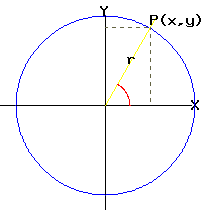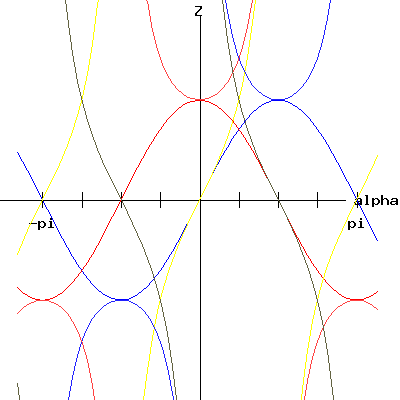Egwald Mathematics: Trigonometry

by

Elmer G. Wiens

Egwald's popular web pages are provided without cost to users.
Please show your support by joining Egwald Web Services as a Facebook Fan:Follow Elmer Wiens on Twitter:Natural and Reciprocal Trigonometric Functionsalpha = the angle between the x-axis and the ray from the origin to the point P. z = sin(alpha) = y / r   z = csc(alpha) = r / y z = cos(alpha) = x / r   z = sec(alpha) = r / x z = tan(alpha) = y / x   z = cot(alpha) = x / y

 sin cos tan csc sec cotCheck the option you want Graph natural trigonometric functions Graph reciprocal trigonometric functions Graph natural and reciprocal trigonometric functionsCopyright © Elmer G. Wiens:   EgwaldWeb ServicesAll Rights Reserved.    Inquiries